Home > A2C > Chapter 11 > Lesson 11.2.6 > Problem11-117

11-117.
1. Which of the following equations is the equation of a hyperbola? How can you tell? What is the shape of the graph of the other equation? After you have decided on the shapes, quickly sketch the graphs. Homework Help ✎

1.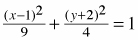2.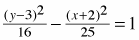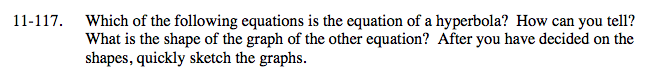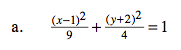This equation has two squared terms that are being added.
Think of this as something like two parabolas being joined.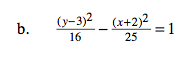This equation has two squared terms that are being subtracted, so one term is positive while the other is negative.
Think of this as something like two parabolas facing opposite directions.